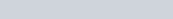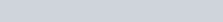ChemTalk

# Osmotic Pressure## Core Concepts

In this article, you will learn about osmotic pressure and how it is calculated. This article will also briefly introduce osmosis and explain how osmotic pressure is used in real world situations

## What is Osmosis?

To understand osmotic pressure, we need some background on osmosis. Osmosis is the simple diffusion of water from a high solute concentration area to a lower solute concentration area. The water pushes through a semi-permeable membrane, which doesn’t allow larger particles through. Osmosis occurs to balance out the concentration of larger particles, or solutes, on two sides of the membrane.

Imagine two rooms at a party, one crowded, and one nearly empty, connected with a abnormally small door. The smaller people, who can fit through the door, start moving from the crowded side to the empty side to give themselves more space. Eventually, the number of people on both sides is the same as people spread out to give everyone even amounts of space. This represents osmosis, where water (the smaller people), moves through a semi-permeable membrane (the door), to balance the concentration of solute (the number of people) on both sides.

Osmosis also takes place naturally and does not require energy, making it a form of passive transport. It occurs in all plants and animals, especially in blood cells.

## What is Osmotic Pressure?

Osmotic pressure is the pressure needed to stop osmosis from occurring. Osmotic pressure is directly proportional to the number of solute molecules present in a solution. Since a greater number of solute molecules means a stronger tendency to balance out, the pressure needed to stop osmosis increases when the number of solute molecules increases. However, osmotic pressure does not depend on the size of the molecules. Even though larger molecules have a greater mass, they have the same number of molecules per mole. Osmosis is also inversely proportional to volume. As long as the number of solute molecules stays the same, a higher volume means a more diluted solution, resulting in less osmosis occurring. Therefore, the pressure needed to stop osmosis increases with the number of solute molecules, and decreases with a greater volume of solvent.

This concept is represented visually by the difference in height between the two sides. The amount of pressure required to stop the movement of H2O from the right side to the left side is the osmotic pressure. Note that because of the membrane(yellow), it is the water that moves to balance out the pressure, not the solute molecules.

## Calculating Osmotic Pressure

Osmotic pressure can be calculated using the formula π = iMRT, provided that “i” is the Van’t Hoff factor, M is the molarity, R is the gas constant, and T is the temperature. The value of R may change depending on what unit of measurements you are using.

To find the Van’t Hoff factor, you need to figure out how many moles of solute will be present in your solution, a.k.a osmoles. This will depend on if and how your solute breaks apart in water. Solutes like glucose (C₆H₁₂O₆) do not break apart, so one mole of glucose will only form one osmole, giving it a Van’t Hoff factor of 1. On the other hand, solutes, like table salt (NaCl), disassociate into one mole of Na+ and Cl. Since there is one mole of each ion, there are two osmoles in total, meaning it has a Van’t Hoff factor of 2.

## Practice Problems

Let’s do some practice! Practice Problems are the best way to quickly get a grasp on challenging topics.

###### Problem 1

What is the Van’t Hoff factor of a solution made with 2.90 moles of CaCl2 and 1.4 moles of (NH4)2SO4 ? Hint: (you don’t need the numbers to solve this problem!)

To do this problem, we need to think about how each of these molecules will break apart, or disassociate in waterRemember, compounds like NH4 and SO4 stay together! If you need a reminder on how ionic compounds disassociate in water, click here!

The numbers given in this problem don’t matter because the molarity of the solution will be included in the equation when finding osmotic pressure. For now, all we are concerned with is the number of molecules in each mole.

Therefore, there is a Van’t Hoff factor of 1 + 2 + 2 + 1 = 6

###### Problem 2

A solution is made with 2.34 moles of sucrose ( C12 H22 O11 ) and 750 mL of water. What is the osmotic pressure at 29 °C?

To solve this problem, we first need to find the molarity of the solution.

2.34 moles / 0.75 mL = 3.12 M solution

Next, we need to determine the osmolarity of the solution, which will tell us the Van’t Hoff factor.

Since sucrose does not disassociate in water, the Van’t Hoff factor is 1.

Now, we can plug in our numbers into the equation π = iMRT. Remember, we need to convert our temperature into Kelvins!

π = 1 • 3.12M • 0.0821 • (29+273) = 77.36 atm

## Clinical Applications of Osmotic Pressure

• Osmotic pressure is constantly referenced when designing IV fluids ; the fluids need to have the proper osmolarity to prevent blood cells from swelling or shrinking
• When treating kidney failure, physicians use a solution with a specific osmolarity to remove waste from the body
• Organs being prepared for transplant are stored in a solution with a specific osmolarity, which preserves the organs for longer outside of the human body
• In severe cases of dehydration, electrolyte solutions with a high osmolarity help to swell red blood cells with the nutrients they need